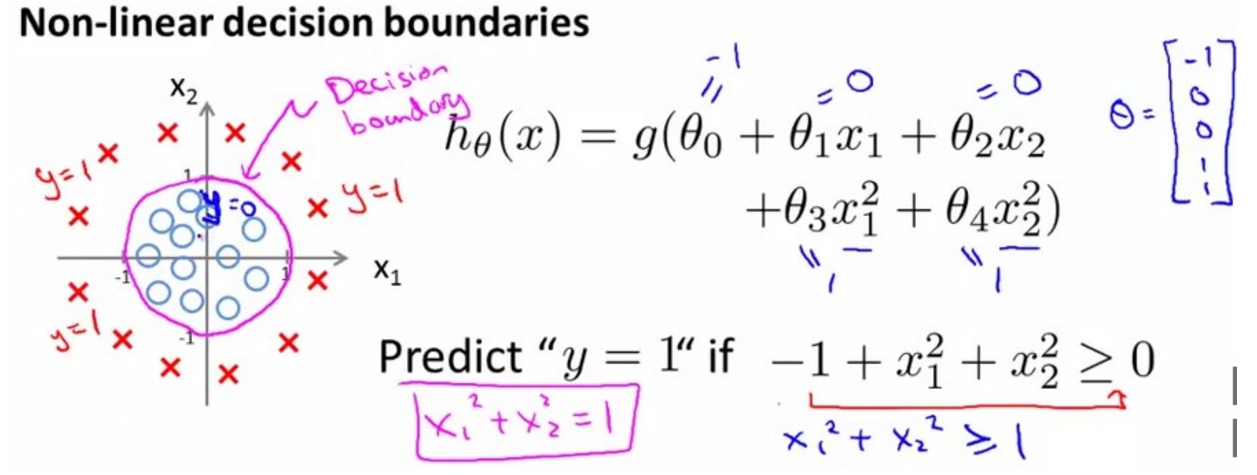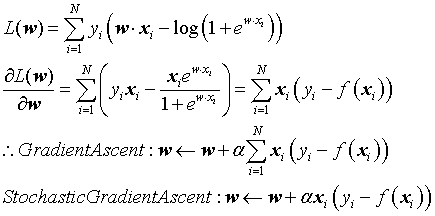# 逻辑斯蒂回归 Logstic Regression in Python

## 1、基本思想

（1）线性回归将输入数据的各个维度的特征进行了有效的结合（通过分配不同的权重），使得所有特征共同协同合作实现对模型的拟合。在此基础上，逻辑斯蒂回归利用sigmoid函数，将特征的线性加权结果映射到0到1之间，而这刚好可以看做是数据样本点属于某一类的概率。如果结果越接近0或者1，说明分类结果的可信度越高。（2）从二项逻辑斯蒂回归模型角度来看，好处是可以将逻辑斯蒂回归模型的二分类问题轻松扩展到多分类问题，具体细节可参阅《统计学习方法》

## 2、注意事项

（1）一般认为，当训练样本数据量足够大，逻辑斯蒂回归将会非常好用，但要注意避免过拟合。

（2）解决过拟合的方法不过两种，一种是减少特征的个数；另一种是模型选择的正则化方法。正则化的话，可以参考岭回归方法。

（3）为了提高分类准确性，在特征选择的时候，由于逻辑斯蒂回归本身的优点，开始的时候不用考虑各个特征之间是否有相关性，直接把能用的特征全部线性加权起来就好。经过初步训练，观察各个特征的权值，如果权值接近为0，那么就可以将这个特征看做是不相关的可以去除的特征。

（4）逻辑斯蒂回归模型最终归结为以似然函数为目标函数的最优化问题，通常通过迭代算法求解，一般采用近似方法如梯度上升法和拟牛顿法。批量梯度上升法由于每次更新回归系数时都需要遍历整个数据集，导致计算量太大，但最终求解的是全局最优解。改进的随机梯度上升法，虽然并不是每次迭代都指向全局最优方向，但大的基本方向是，最终结果往往在全局最优解附近。牛顿法或拟牛顿法一般收敛速度更快。（5）随机梯度上升法需要注意两个细节。

（6）其实，逻辑斯蒂回归模型可以看做由两部分组成。一部分跟线性回归一样，就是一条直线；另一部分就是sigmoid函数。需要注意的是，有了权重向量我们就可以线性加权了，我们的直线就是令线性加权的结果为0的公式表达。为什么要人为定为0呢？当然是为了sigmod函数啊。这样才能使正样本的线性加权大于0，在sigmod中接近1，同样负样本的线性加权小于0，在sigmod中接近0。在SVM中，同样也是先学习一个分离超平面，然后带入分类决策函数sign中。

（7）线性感知机直接用线性回归做分类，是因为考虑到了所有样本点到分类决策面的距离，所以在两类数据分布不均匀的时候将导致误差非常大；逻辑斯蒂回归和SVM克服了这个缺点，前者采用将所有数据采用sigmoid函数进行了非线性映射，使得远离分类决策面的数据作用减弱；后者则直接去掉了远离分类决策面的数据，只考虑支持向量的影响。

## 5、Python实现

from numpy import *

### preparing data ###
def loadDataSet():
dataMat = []
labelMat = []
fr = open(r'F:\ResearchData\MyCode\Python\logRegres\testSet.txt')
for line in fr.readlines():
lineArr = line.strip().split()
dataMat.append([1.0, float(lineArr), float(lineArr)])
labelMat.append(int(lineArr))
return dataMat, labelMat

### training ###
def sigmoid(inX):
return 1.0/(1+exp(-inX))

# gradient ascent methond
def gradAscent(dataMatIn, classLabels):
dataMatrix = mat(dataMatIn)
labelMat = mat(classLabels).transpose()
N, n = shape(dataMatrix)
alpha = 0.001
maxItera = 500
weights = ones((n, 1))
for kk in range(maxItera):
f = sigmoid(dataMatrix*weights)
error = labelMat - f
weights = weights + alpha * dataMatrix.transpose() *error
return weights

# stochastic gradient ascent method
def stocGradAscentV1(dataMatrix, classLabels):
N, n = shape(dataMatrix)
alpha = 0.01
weights = ones(n)
for ii in range(N):
f = sigmoid(sum(dataMatrix[ii]*weights))
error = classLabels[ii] - f
weights = weights + alpha*error*dataMatrix[ii]
return weights

def stocGradAscentV2(dataMatrix, classLabels, numIter=150):
N, n = shape(dataMatrix)
weights = ones(n)
for ii in range(numIter):
dataIndex = range(N)
for nn in range(N):
alpha = 4/(1.0+ii+nn) + 0.01
randIndex = int(random.uniform(0, len(dataIndex)))
f = sigmoid(sum(dataMatrix[randIndex]*weights))
error = classLabels[randIndex] - f
weights = weights + alpha*error*dataMatrix[randIndex]
del(dataIndex[randIndex])
return weights

# plotting
def plotBestFit(weights):
import matplotlib.pyplot as plt
dataMat, labelMat = loadDataSet()
dataArr = array(dataMat)
n = shape(dataArr)
xcord1 = []
ycord1 = []
xcord2 = []
ycord2 = []
for ii in range(n):
if int(labelMat[ii]) == 1:
xcord1.append(dataArr[ii, 1])
ycord1.append(dataArr[ii, 2])
else:
xcord2.append(dataArr[ii, 1])
ycord2.append(dataArr[ii, 2])
fig = plt.figure()
ax = fig.add_subplot(111)
ax.scatter(xcord1, ycord1, s=30, c='red', marker='s')
ax.scatter(xcord2, ycord2, s=30, c='green')
x = arange(-3.0, 3.0, 0.1)
y = (-weights-weights*x)/weights
ax.plot(x, y)
plt.xlabel('feature1')
plt.ylabel('feature2')
plt.show()

### testing ###
def classifyVector(inX, weights):
prob = sigmoid(sum(inX*weights))
if prob > 0.5:
return 1.0
else:
return 0.0

def colicTest():
frTrain = open(r'F:\ResearchData\MyCode\Python\logRegres\horseColicTraining.txt')
frTest = open(r'F:\ResearchData\MyCode\Python\logRegres\horseColicTest.txt')
trainingSet = []
trainingLabels = []
for line in frTrain.readlines():
currLine = line.strip().split('\t')
lineArr = []
for ii in range(21):
lineArr.append(float(currLine[ii]))
trainingSet.append(lineArr)
trainingLabels.append(float(currLine))
trainWeights = stocGradAscentV2(array(trainingSet), trainingLabels, 500)
errorCount = 0
numTestVec = 0.0
for line in frTest.readlines():
numTestVec += 1.0
currLine = line.strip().split('\t')
lineArr = []
for ii in range(21):
lineArr.append(float(currLine[ii]))
if int(classifyVector(array(lineArr), trainWeights)) != int(currLine):
errorCount =+ 1
errorRate = (float(errorCount)/numTestVec)
print "the error rate of this test is: %f" % errorRate
return errorRate

def multiTest():
numTests = 10
errorSum = 0.0
for kk in range(numTests):
errorSum += colicTest()
print "after %d iterations, the average error rate is: %f" % (numTests, errorSum/float(numTests))08-07
01-104040
11-061665
05-073219
01-091712
09-08557
12-05250
10-30821
09-184747
07-271497
09-075062
07-23959
12-21161
09-02652
07-28689
©️2020 CSDN 皮肤主题: Age of Ai 设计师:meimeiellie